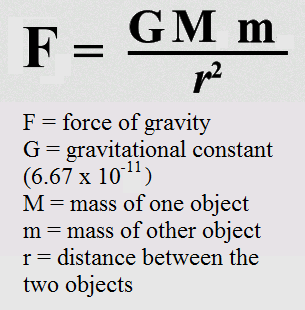Gravity, or gravitation, is a natural phenomenon by which all things with mass are brought toward (or gravitate toward) one another, including planets, stars and galaxies. Since energy and mass are equivalent, all forms of energy, including light, also cause gravitation and are under the influence of it. On Earth, gravity gives weight to physical objects and causes the ocean tides. The gravitational attraction of the original gaseous matter present in the Universe caused it to begin coalescing, forming stars — and the stars to group together into galaxies — so gravity is responsible for many of the large scale structures in the Universe. Gravity has an infinite range, although its effects become increasingly weaker on farther objects.

Gravity is the weakest of the four fundamental interactions of nature. As a consequence, gravity has a negligible influence on the behavior of subatomic particles, and plays no role in determining the internal properties of everyday matter. On the other hand, gravity is the dominant interaction at the macroscopic scale, and is the cause of the formation, shape and trajectory (orbit) of astronomical bodies. It is responsible for various phenomena observed on Earth and throughout the Universe; for example, it causes the Earth and the other planets to orbit the Sun, the Moon to orbit the Earth, the formation of tides, the formation and evolution of the Solar System, stars and galaxies.

## Newton’s Law of Gravitation

Gravitational force is a attractive force between two masses m1 and m2 separated by a distance r. The gravitational force acting between two point objects is proportional to the product of their masses and inversely proportional to the square of the distance between them.The value of G is 6.67 X 10-11 Nm2 kg-2 and is same throughout the universe. The value of G is independent of the nature and size of the bodies well as the nature of the medium between them. Dimensional formula of G is [M-1L3T-2].

### Important Points about Gravitation Force

• Gravitational force is a central as well as conservative force.
• It is the weakest force in nature.
• It is 1036 times smaller than electrostatic force and 1018 times smaller than nuclear force.
• The law of gravitational is applicable for all bodies, irrespective of their size, shape and position.
• Gravitational force acting between sun and planet provide it centripetal force for orbital motion.
• Gravitational pull of the earth is called gravity.
• Newton’s third law of motion holds good for the force of gravitation. It means the gravitation forces between two bodies are action-reaction pairs.

### Following three points are important regarding the gravitational force

(i) Unlike the electrostatic force, it is independent of the medium between the particles.

(ii) It is conservative in nature.

(iii) It expresses the force between two point masses (of negligible volume). However, for external points of spherical bodies the whole mass can be assumed to be concentrated at its centre of mass.

Note: Newton’s law of gravitation holds goods for object lying at very large distances and also at very short distances. It fails when the distance between the objects is less than 10-9 m i.e., of the order of intermolecular distances.

## Acceleration Due to Gravity

The uniform acceleration produced in a freely falling object due to the gravitational pull of the earth is known as acceleration due to gravity. It is denoted by g and its unit is m/s2. The value of g is independent of the mass of the object which is falling freely under gravity. The value of g changes slightly from place to place but is taken to be 9.8 m/s2 for all practical purposes.

The value of acceleration due to gravity on the moon is about one sixth of that on the earth and on the sun is about 27 times of that on the earth. Among the planets, the acceleration due to gravity is minimum on the mercury.

It is given by g = GM / R2 ; where M = mass of the earth = 6.0 * 1024 kg and R = radius of the earth = 6.38 * 106 m.

Acceleration due to gravity at a height h above the surface of the earth is given by

gh = GM / (R+h)2 = g (1 – 2h / R)

### Factors Affecting Acceleration Due to Gravity

(i) Shape of Earth Acceleration due to gravity g varies as 1 / R2. Earth is elliptical in shape. Its diameter at poles is approximately 42 km less than its diameter at equator. Therefore, g is minimum at equator and maximum at poles.

(ii) Rotation of Earth about Its Own Axis If ω is the angular velocity of rotation of earth about its own axis, then acceleration due to gravity at a place having latitude λ is given by

g’ = g – Rω2 cos2 λAt poles λ = 90° and g’ = g. Therefore, there is no effect of rotation of earth about its own axis at poles.

At equator λ = 0° and g’ = g – Rω2. The value of g is minimum at equator. If earth stops its rotation about its own axis, then g will remain unchanged at poles but increases by Rω2 at equator.

(iii) Effect of Altitude The value of g at height h from earth’s surface

Therefore g decreases with altitude.

(iv) Effect of Depth The value of gat depth h from earth’s surface

g’ = g * (1 – h / R)

Therefore g decreases with depth from earth’s surface. The value of g becomes zero at earth’s centre.

That’s all in gravitation for now. You can brush up your concepts on Friction here. Till then keep shining & All the best!

Access 300,000+ questions curated by India’s top rankers.

+91
No thanks.

## Request a Free 60 minute counselling session at your home

Please enter a valid phone number
•7,829,648

Happy Students
•358,177,393

Questions Attempted
•3,028,498

Tests Taken
•3,020,367